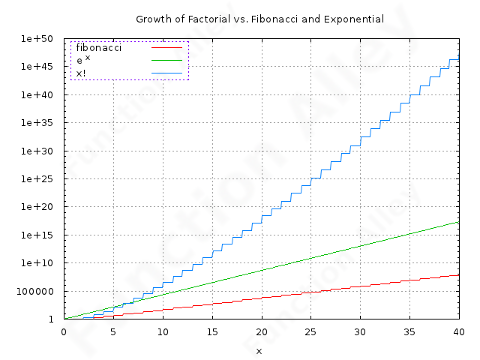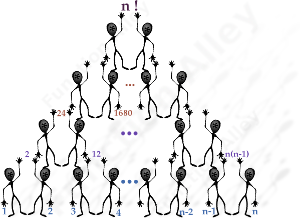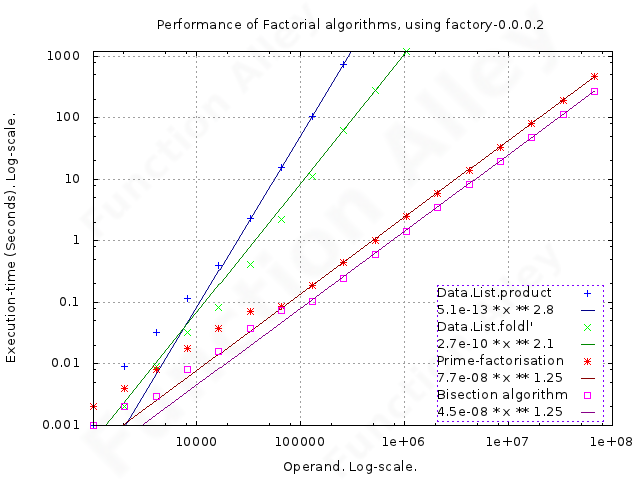# Factorials !The factorial of a positive integer n (denoted n!), is merely the product of all positive integers less than or equal to n.

Before your brain has the chance to say "Is that all there is", this rabbit-hole goes a little deeper. The factorial function grows faster than any exponential (notice the log-linear scale on the graph). Because of this, the largest integer, for which one may compute the factorial, using a 32-bit integer, is an unimpressive 12, & by trading-up to 64 bits, just 20 (unsigned arithmetic doesn't help); thus unbounded integer-arithmetic is required.

## Algorithms

The Bisection Algorithm:

"I was thinking of a way to multiply by ten, and always, in the answer, get the question back again."The next issue is the efficiency of the requisite multiplications. Integer-multiplication normally has O(n2) time-complexity, but the exponent of this can be reduced significantly using, for example, the Karatsuba algorithm. This multiplication-algorithm, & other similar ones, only pay dividends when the operands exceed a minimum magnitude.

Naïvely performing the (n − 1) multiplications sequentially, results in the multiplication of a small integer by a huge integer, but by bisecting the list, & multiplying the recursively evaluated halves (which requires the same total number of multiplications), results in operands of similar magnitude, permitting utilisation of these superior multiplication-algorithms. One doesn't need to implement any fancy multiplication-algorithm, rather just create the conditions under which the underlying library-function can utilise whatever P2C2E has already been implemented for large integers.

Prime-factorisation:

"The Times They Are A-Changin'."

Because the prime factors of n! are all less than or equal to n, one can avoid the drudgery of multiplying the sequence of integers from which the factorial is composed, & arrive at the same result, from the product of the relatively small number of prime factors. Normally prime-factorisation (having exponential time-complexity), would be too expensive to be considered as a potential time-saving mechanism, but because of the predictable structure of factorials, one can extract the prime factors efficiently using the Legendre Theorem.

## Testing

My usual test-platform was used.

Though the divide-and-conquer strategy looks ripe for parallelization, I observed no improvement, when using both cores of my 2-core hardware. This is probably because the execution-time is dominated by the final multiplication at the apex of the pyramid, where there are no gains to be made from parallelization, leaving only the inevitable loss from thread-synchronisation.## Results

I've fitted polynomials to the performance-data for each algorithm, so that one can estimate their time-complexity. Because of the log-log plot, these polynomials appear as straight lines.

• Performing the multiplications sequentially, using Haskell's convenient lazy function "Data.List.product", results in disastrous performance, with nearly O(n3) time-complexity.

• Replacing this with the strict equivalent,
``Data.List.foldl' (*) 1 [2 .. n]``
results in a marked improvement, reducing the time-complexity to O(n2).
• Use of the bisection-algorithm, reduced the time-complexity to nearly O(n).

• Prime-factorisation, neither improved on the results already obtained by simpler means (within the domain of integers tested), nor did the apparent time-complexity suggest that it would for larger integers.

## Conclusions

Use of the standard function "Data.List.product", results in the unnecessary overhead of lazy-evaluation. The staggering extent of the improvement resulting from replacing sequential multiplication with the bisection-algorithm, can be understood when one assimilates the magnitude of the integers being multiplied (106! has 5,565,709 digits; even if the last 249,998 of them are zero), & therefore the potential dividend from efficient multiplication.

The performance of the prime-factorisation algorithm was disappointing, but because the performance was close to that obtained from the bisection-algorithm, further refinement of the implementation may yet make it competitive. Products & ratios of factorials are frequently encountered (eg. the Taylor-series for square-roots, & Pi-formulae by Ramanujan, Chudnovsky & Borwein), & whilst any simple ratio of factorials (ie. x! ÷ y!) can easily be reduced, by cancelling all the integers from which the smaller of the two factorials is composed, to a form a single rising factorial, more complex combinations (eg. x! ÷ (y! × z!)), might be efficiently tackled by prime-factorisation, which facilitates cancellation of common terms, by mere addition & subtraction of the exponents to which the prime factors are raised. So, this algorithm may yet have a niche.